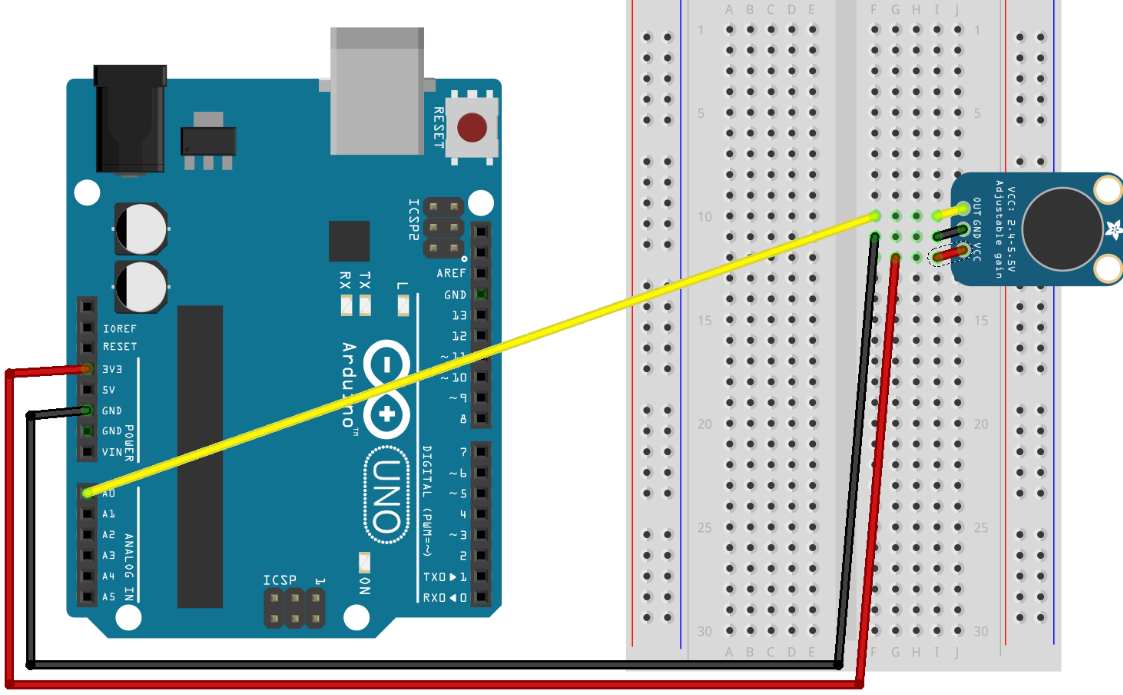# Electret Microphone Amplifier

## Electret Microphone Amplifier### Components

```1 x electret microphone (Adafruit MAX4466)
1 x Arduino Uno
1 x breadboard
jumper wires
```

### Setup### Arduino sketch

Inspect Serial monitor (9600) for sensor values (converted to volts).
```/*
MAX4466 (adafruit mic)

Written by Shani Mensing, edited by Audrey St. John

Circuit: microphone VCC connected to 3.3V, OUT to analog input A0
*/

// hook up the out of the mic to analog input A0
#define MIC_IN A0

// Sample window width in milliseconds (50 ms = 20Hz)
int sampleWindow = 50;

/**
* Initialization code
**/
void setup()
{
// open serial port and set data rates to 9600 bps (bits-per-second)
// this lets us communicate to/from the arduino
Serial.begin(9600);

pinMode( MIC_IN, INPUT );
}

/**
* Main program loop happens constantly.
**/
void loop()
{
// read the analog sensor as volts
double soundSensed = sampleSoundPeak();

// convert to volts
double volts = (soundSensed * 3.3) / 1024;

// print it out
Serial.println(volts);
}

/////////////// Our own methods

/**
* Sense biggest input difference are being input from the analog MIC sensor
* over a certain "window" of time.
* Values returned are in the range 0 - 1024.
**/
double sampleSoundPeak()
{
// record start time
double startMillis = millis();

// this will be the highest peak, so start it very small
int signalMax = 0;

// this will be the lowest peak, so start it very high
int signalMin = 1024;

// will hold the current value from the microphone
int sample;

// collect data for 50 ms
while ( (millis() - startMillis) < sampleWindow )
{
// read a value from mic and record it into sample variable
sample = analogRead( MIC_IN );

// toss out spurious readings
if (sample < 1024)
{

// if the current sample is larger than the max
if (sample > signalMax)
{
// this is the new max -- save it
signalMax = sample;
}
// otherwise, if the current sample is smaller than the min
else if (sample < signalMin)
{
// this is the new min -- save it
signalMin = sample;
}
}
}

// now that we've collected our data,
// determine the peak-peak amplitude as max - min
int peakDifference = signalMax - signalMin;

// give it back to the caller of this method
return peakDifference;
}

```